## GATE 2008-IT

 Question 1

A set of Boolean connectives is functionally complete if all Boolean functions can be synthesized using those. Which of the following sets of connectives is NOT functionally complete?

 A EX-NOR B implication, negation C OR, negation D NAND
Digital-Logic-Design       Boolean-Functions
Question 1 Explanation:
→ EX-NOR is not functionally complete.
→ NOR and NAND are the functionally complete logic gates, OR, AND, NOT only logic gate can be implemented by using them.
→ And (Implication, Negation) is also functionally complete.
 Question 2

A sample space has two events A and B such that probabilities P(A ∩ B) = 1/2, P(A') = 1/3, P(B') = 1/3. What is P(A ∪ B)?

 A 11/12 B 10/12 C 9/12 D 8/12
Engineering-Mathematics       Probability
Question 2 Explanation:
P(A ∩ B) = 1/2
P(A') = 1/3; P(A) = 2/3
P(B') = 1/3; P(B) = 2/3
P(A ∪ B) = P(A) +P(B) - P(A ∩ B)
= 2/3 + 2/3 - 1/2
= 4+4-3/ 6
= 5/6
= 10/12
 Question 3

What is the chromatic number of the following graph?A 2 B 3 C 4 D 5
Engineering-Mathematics       Graph-Theory
Question 3 Explanation:
Chromatic number = 3
→ Chromatic number of a graph is the smallest number of colours needed to colour the vertices so that no two adjacent vertices share the same colour.Question 4

What is the size of the smallest MIS(Maximal Independent Set) of a chain of nine nodes?

 A 5 B 4 C 3 D 2
Engineering-Mathematics       Graph-Theory
Question 4 Explanation:
1 - 2 - 3 - 4 - 5 - 6 - 7 - 8 - 9
(2, 5, 8) is the maximal independent set for a chain of 9 nodes. If we add any start node to the set then it will not be MIS.
Independent set:
A set of vertices is called independent set such that no two vertices in the set are adjacent.
 Question 5

Which of the following regular expressions describes the language over {0, 1} consisting of strings that contain exactly two 1’s?

 A (0 + 1) * 11(0 + 1) * B 0 * 110 * C 0 * 10 * 10 * D (0 + 1) * 1(0 + 1) * 1 (0 + 1) *
Theory-of-Computation        Regular-Expressions
Question 5 Explanation:
Option A: (0+1)* 11(0+1)*
→ 1110 this consists of 3 ones but accepted by given expression. So this is false.
Option B: 0* 110*
→ 0101 this string consists of two is but not accepted by given expression. This is false.
Option C: 0* 10* 10*
→ This is true.
Option D: (0+1)* 1(0+1)* 1(0+1)*
→ 011010 - This can have three is but accepted by given expression. So this is also false.
 Question 6

Let N be an NFA with n states and let M be the minimized DFA with m states recognizing the same language. Which of the following in NECESSARILY true?

 A m ≤ 2n B n ≤ m C M has one accept state D m = 2n
Theory-of-Computation       Finite-Automata
Question 6 Explanation:
Set of states of NFA = n
A state in a DFA is a proper subset of states of NFA of corresponding DFA.
→ No. of subsets with n elements = 2n
→ m ≤ 2n
 Question 7

The following bit pattern represents a floating point number in IEEE 754 single precision format

` 110000011101000000000000000000000`
The value of the number in decimal form is
 A -10 B -13 C -26 D None of these
Digital-Logic-Design       Number-Systems
Question 7 Explanation:
Sign bit is 1 then given number is negative.
Exponent bits - 10000011
Exponent can be added with 127 bias in IEEE single precision format then outval exponent
= 10000011 - 127
= 131 - 127
= 4
→ In IEEE format, an implied 1 is before mantissa, and hence the outval number is
→ 1.101 × 24 = -(11010)2 = -26
 Question 8

Consider the following Boolean function of four variables

` f(A,B,C,D) = Σ(2, 3, 6, 7, 8, 9, 10, 11, 12, 13)`
The function is
 A independent of one variable B independent of two variables C independent of three variable D dependent on all the variables
Digital-Logic-Design       Boolean-Functions
Question 8 Explanation:
f(A, B, C, D) = Σ(2, 3, 6, 7, 8, 9, 10, 11, 12, 13)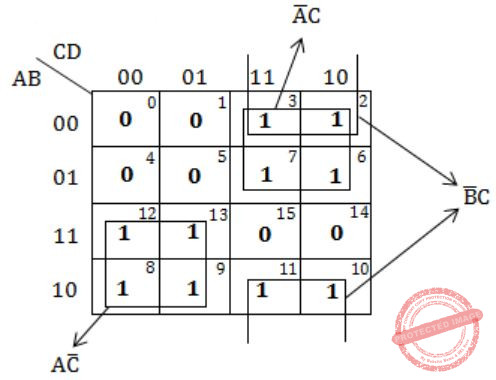Independent of one variable '0'.
 Question 9

What Boolean function does the circuit below realize ?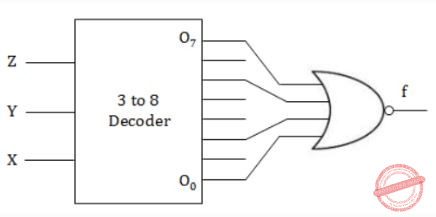A xz+x’z’ B xz’+x’z C x’y’+yz D xy+y’z’
Digital-Logic-Design       Decoder
Question 9 Explanation:
f = (x’y’z’ + x’yz’+xy’z+xyz)’
= (x’z’ + xz)’
= x’z + xz’
 Question 10

Arrange the following functions in increasing asymptotic order:

```A. n1/3
B. en
C. n7/4
D. n log9n
E. 1.0000001n```
 A A, D, C, E, B B D, A, C, E, B C A, C, D, E, B D A, C, D, B, E
Algorithms        Time-Complexity
Question 10 Explanation:
A < D < C < E < B
n1/3 < n log9 < n7/4 < 1.0000001n < en
 Question 11

For problems X and Y, Y is NP-complete and X reduces to Y in polynomial time. Which of the following is TRUE?

 A If X can be solved in polynomial time, then so can Y B X is NP-complete C X is NP-hard D X is in NP, but not necessarily NP-complete
Algorithms        P-NP
Question 11 Explanation:
Note: Out of syllabus.
 Question 12

Which of the following is TRUE?

 A The cost of searching an AVL tree is θ (log n) but that of a binary search tree is O(n) B The cost of searching an AVL tree is θ (log n) but that of a complete binary tree is θ (n log n) C The cost of searching a binary search tree is O (log n) but that of an AVL tree is θ(n) D The cost of searching an AVL tree is θ (n log n) but that of a binary search tree is O(n)
Data-Structures       AVL-Trees
Question 12 Explanation:
Complexity of AVL tree is O(logn) because it is a balanced tree, in Worst case binary search tree complexity is O(n).
 Question 13

Match the programming paradigms and languages given in the following table.A I-c, II-d, III-b, IV-a B I-a, II-d, III-c, IV-b C I-d, II-c, III-b, IV-a D I-c, II-d, III-a, IV-b
Programming       Match-the-Following
Question 13 Explanation:
Note: Out of syllabus.
 Question 14

Consider the execution of the following commands in a shell on a linux operating system.

```system
bash\$cat alpha
Mathematics
bash\$in alpha beta
bash\$ rm alpha
bash\$cat >> beta << SAME
Information Technology
SANE
bash\$ cat beta```
The output of the last command will be:
 A Mathematics Information Technology SAME. B Mathematics Information Technology. C Information Technology. D Information Technology SAME.
Operating-Systems       LINUX
Question 14 Explanation:
Note: Out of syllabus.
 Question 15

A processor that has carry, overflow and sign flag bits as part of its program status word (PSW) performs addition of the following two 2's complement numbers 01001101 and 11101001. After the execution of this addition operation, the status of the carry, overflow and sign flags, respectively will be:

 A 1, 1, 0 B 1, 0, 0 C 0, 1, 0 D 1, 0, 1
Digital-Logic-Design       Number-Systems
Question 15 Explanation:Carry flag = 1
Overflow flag = 0
Sign bit = 0 (MSB bit is 0)
Overflow flag:
In computer processors, the overflow flag is usually a single bit in a system status register used to indicate when an arithmetic overflow has occurred in an operation.
 Question 16

A paging scheme uses a Translation Look-aside Buffer (TLB). A TLB-access takes 10 ns and a main memory access takes 50 ns. What is the effective access time(in ns) if the TLB hit ratio is 90% and there is no page-fault?

 A 54 B 60 C 65 D 75
Operating-Systems        Virtual Memory
Question 16 Explanation:
EMAT = TLB access time + Miss ratio × Main memory access time + Main memory access time
= 10 + 0.1 × 50 + 50
= 10 + 5 + 50
= 65
 Question 17

Find if the following statements in the context of software testing are TRUE or FALSE.
(S1) Statement coverage cannot guarantee execution of loops in a program under test.
(S2) Use of independent path testing criterion guarantees execution of each loop in a program under test more than once.

 A True, True B True, False C False, True D False, False
Question 17 Explanation:
Note: Out of syllabus.
 Question 18

How many bytes of data can be sent in 15 seconds over a serial link with baud rate of 9600 in asynchronous mode with odd parity and two stop bits in the frame?

 A 10,000 bytes B 12,000 bytes C 15,000 bytes D 27,000 bytes
Computer-Organization       Serial-Communication
Question 18 Explanation:
In the Asynchronous mode of transmission along with per byte, we need to send some extra bit like start, stop bit and parity bit, etc.
1 bit for start bit, 8 bits for data, 1 bit for parity, 2 bits for stop bits i.e.,Question 19

Which of the following is TRUE only of XML but NOT HTML?

 A It is derived from SGML B It describes content and layout C It allows user defined tags D It is restricted only to be used with web browsers
Question 19 Explanation:
Note: Out of syllabus.
 Question 20

Provider the best matching between the entries in the two columns given in the table below:A I-a, II-d, III-c, IV-b B I-b, II-d, III-c, IV-a C I-a, II-c, III-d, IV-b D I-b, II-c, III-d, IV-a
Computer-Networks       Match-the-Following
Question 20 Explanation:
(i) Proxy server: Proxy server and firewall are to be combined.
(ii) Kazaa and DC++ are P2P applications.
(iii) P2P slip is a processor of PPP.
(iv) DNS allows caching of entries at local server.
 Question 21

Which of the following first order formula is logically valid? Here α(x) is a first order formula with x as a free variable, and β is a first order formula with no free variable.

 A [β→(∃x,α(x))]→[∀x,β→α(x)] B [∃x,β→α(x)]→[β→(∀x,α(x))] C [(∃x,α(x))→β]→[∀x,α(x)→β] D [(∀x,α(x))→β]→[∀x,α(x)→β]
Engineering-Mathematics       Propositional-Logic
Question 21 Explanation:
[(∃x,α(x))→β]→[∀x,α(x)→β]
L.H.S. : If there is an x such that α(x) is true, then β is true.
R.H.S. : For all x, if α(x) true, then β is true.
Here, the given LHS and RHS are to be same as β is a formula which can be independent of x (if β is true for one x, it is true for every x, and vice-versa).
Here, LHS = RHS
So, Option C is valid.
 Question 22

Which of the following is the negation of [∀x, α →(∃y, β →(∀ u, ∃v, y))]

 A [∃x, α → (∀y, β → (∃u, ∀v, y))] B [∃x, α → (∀y, β → (∃u, ∀ v, ¬y))] C [∀x, ¬α → (∃y, ¬β → (∀u, ∃v, ¬y))] D [∃x, α ʌ (∀y, β ʌ (∃u, ∀v, ¬y))]
Engineering-Mathematics       Propositional-Logic
Question 22 Explanation:
~[∀x, α → (∃y, β → (∀u, ∃v, y))]
⇔ [∃x, [α × ~(∃y, β → (∀u, ∃v, y))]
⇔ [∃x, [α × ∀y, ~(β → (∀u, ∃v, y)]
⇔ [∃x, [α × ∀y, ~(β × ~(∀u, ∃v, y)]
⇔ [∃x, [α × ∀y(β × (∃u, ∀v, y)]
 Question 23

What is the probability that in a randomly chosen group of r people at least three people have the same birthday?

 ABCDEngineering-Mathematics       Probability
Question 23 Explanation:Question 24

The exponent of 11 in the prime factorization of 300! is

 A 27 B 28 C 29 D 30
Engineering-Mathematics       Combinatorics
Question 24 Explanation: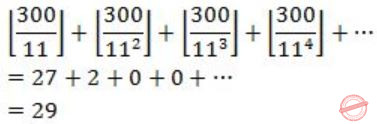Question 25

In how many ways can b blue balls and r red balls be distributed in n distinct boxes?

 ABCD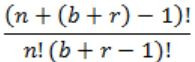Engineering-Mathematics       Combinatorics
Question 25 Explanation:
No. of blue ball distributed to n boxes in
C(n+b-1, b) = (n+b-1)!/ (n-1)!b!
No. of red distributed to n boxes is
(n+r-1, r) = (n+r-1)!/ (n-1)!r!
Total no. of ways = (n+b-1)!/(n-1)!b! * (n+r-1)!/(n-1)!r!
= (n+b-1)!(n+r-1)!/(n-1)!b!(n-1)!r!
 Question 26

Consider the field C of complex numbers with addition and multiplication. Which of the following form(s) a subfield of C with addition and multiplication?

```(S1) the set of real numbers
(S2) {(a+ib) | a and b are rational numbers}
(S3) {a+ib | (a2 + b2) ≤ 1}
(S4) {ia | a is real}```
 A only S1 B S1 and S3 C S2 and S3 D S1 and S2
Engineering-Mathematics       Sets and Relations
Question 26 Explanation:
S1: It is closed under both * and +.
As real + real = real and real * real = real
S2: It is closed as rational + rational = rational and rational * rational = rational
S3: It is not closed.
⇒ (0.3+0.4i) + (0.7+0.6i) = 1+i
Both (0.3+0.4i) & (0.7+0.6i) are complex numbers follows S3 but addition of them doesn't follows.
⇒ 1+i, (a2+b2) <= 1
⇒ 1+1 is not less than or equal to 1.
S4: {ia | a ia real}
In this there is no multiplicative identity exists (i.e., 1).
 Question 27

G is a simple undirected graph. Some vertices of G are of odd degree. Add a node v to G and make it adjacent to each odd degree vertex of G. The resultant graph is sure to be

 A Regular B Complete C Hamiltonian D Euler
Engineering-Mathematics       Graph-Theory
Question 27 Explanation:
Euler graph theory is a trail in a finite graph which visits every edge exactly once and which is a undirected graph.
→ In Euler graph all degrees must be even for all nodes. And number of odd degree vertices should be even.
→ So, degree of this new node will be even and as a new edge is formed between this new node and all other nodes of odd degree hence here is not a single node exists with degree odd so this is Euler graph.
 Question 28

Consider the following Hasse diagrams.A (i) and (iv) only B (ii) and (iii) only C (iii) only D (i), (ii) and (iv) only
Engineering-Mathematics       Sets-And-Relation
Question 28 Explanation:
If a hasse diagram is a lattice then every pair of elements will have exactly one lub and gub.
(ii) and (iii), having more than one lub or glb for some pairs due to which they are not lattice.
 Question 29

If M is a square matrix with a zero determinant, which of the following assertion(s) is(are) correct?

• (S1) Each row of M can be represented as a linear combination of the other rows

• (S2) Each column of M can be represented as a linear combination of the other columns

• (S3) MX = 0 has a nontrivial solution

(S4) M has an inverse
 A S3 and S2 B S1 and S4 C S1 and S3 D S1, S2 and S3
Engineering-Mathematics       Linear-Algebra
Question 29 Explanation:
It is given that determinant of square matrix M is zero, means rows of M can be represented as a linear combination of other rows or columns of M can be represented as a linear combination of other columns. Also if determinant of matrix is zero then rank of matrix, which means MX=0 have infinite no.of solution or non – trivial solution.
If determinant of some square matrix is zero then matrix do not have any inverse.
Hence, from the above definitions we can conclude that S1, S2, S3 are true and S4 is false.
 Question 30

Consider the function f(x) = x2 - 2x - 1. Suppose an execution of the Newton-Raphson method to find a zero of f(x) starts with an approximation x0 = 2 0f x. What is the value of x2, 'the approximation of x' that the algorithm produces after two iterations, rounded to three decimal places?

 A 2.417 B 2.419 C 2.423 D 2.425
Engineering-Mathematics       Newton-Raphson-Method
Question 30 Explanation:
Note: Out of syllabus.
 Question 31

If f(x) is defined as follows, what is the minimum value of f(x) for x∊(0,2] ?A 2 B 2(1/12) C 2(1/6) D 2(1/2)
Engineering-Mathematics       Calculus
Question 31 Explanation:
If x = 3/2
f(x) = 25/8x = 25/8(3/2) = 25/12 = 2(1/12)
 Question 32

If the final states and non-final states in the DFA below are interchanged, then which of the following languages over the alphabet {a,b} will be accepted by the new DFA?A Set of all strings that do not end with ab B Set of all strings that begin with either an a or a b C Set of all strings that do not contain the substring ab D The set described by the regular expression b*aa*(ba)*b*
Theory-of-Computation       Finite-Automata
Question 32 Explanation:Option B: abab is not accepted by given RE.
Option C: aba is accepted by given RE.
Option D: ab is not accepted by RE and it belongs to b*aa*(ba)*b*.
 Question 33

Consider the following languages.

```L1 = {ai bj ck | i = j, k ≥ 1}
L1 = {ai bj | j = 2i, i ≥ 0}```
Which of the following is true?
 A L1 is not a CFL but L2 is B L1 ∩ L2 = ∅ and L1 is non-regular C L1 ∪ L2 is not a CFL but L2 is D There is a 4-state PDA that accepts L1, but there is no DPDA that accepts L2
Theory-of-Computation       Identify-Class-Language
Question 33 Explanation:
→ Both L1 and L2 are CFL. So option A is false.
→ L1 ∩ L2 = ∅, True and also L1 is non-regular. Option B is true.
→ L1 ∪ L2 is not a CFL but L2 is CFL is closed under union. So option C is false.
→ Both L1 and L2 accepted by DPDA.
 Question 34

Consider a CFG with the following productions.

```S → AA | B
A → 0A | A0 | 1
B → 0B00 | 1```
S is the start symbol, A and B are non-terminals and 0 and 1 are the terminals. The language generated by this grammar is
 A {0n 102n | n ≥ 1} B {0i 10j 10k | i, j, k ≥ 0} ∪ {0n 102n | n ≥ l} C {0i 10j | i, j ≥ 0} ∪ {0n 102n | n ≥ l} D The set of all strings over {0, 1} containing at least two 0’s E None of the above
Theory-of-Computation       Contest-Free-Grammar
Question 34 Explanation:
S → AA | B
A → 0A | A0 | 1
B → 0B00 | 1
In this B → 0B00 | 1 which generates {0n 102n | n ≥0}
S → AA | B
A → 0A | A0 | 1
Which generates 0A0A → 00A0A → 00101.
Which is suitable for B and D option. D is not correct because 00 is not generated by the given grammar. So only option B is left. Non-terminal B i s generating the second part of B choice and AA is generating the first part.
{0i 10j 10k | i, j, k ≥ 0} ∪ {0n 102n | n ≥ 0}
 Question 35

Which of the following languages is (are) non-regular? L1 = {0m1n | 0 ≤ m ≤ n ≤ 10000} L2 = {w | w reads the same forward and backward} L3 = {w ∊ {0, 1} * | w contains an even number of 0's and an even number of 1's}

 A L2 and L3 only B L1 and L2 only C L3 only D L2 only
Theory-of-Computation       Identify-Class-Language
Question 35 Explanation:
L1 and L3 are regular.
L1 is limited to fixed range and L3 contains even number of 0's which is regular. No need to use more memory to implement L3.
 Question 36

Consider the following two finite automata. M1 accepts L1 and M2 accepts L2.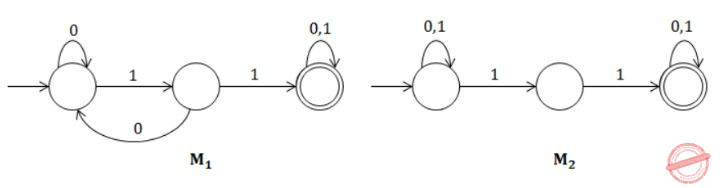Which one of the following is TRUE?
 A L1 = L2 B L1 ⊂ L2 C L1 ∩ L2‘ = ∅ D L1 ∪ L2 ≠ L1 E A and C
Theory-of-Computation       Finite-Automata
Question 36 Explanation:
In this L1 = (0+10)* 11(0+1)*
L2 = (0=1)* 11(0+1)*
Both L1 and L2 are equal.
Option A is correct.
→ L1 ∩ L2‘ = L1 ∩ L1‘ = ∅ (option C also correct)
 Question 37

Consider the following state diagram and its realization by a JK flip flopThe combinational circuit generates J and K in terms of x, y and Q. The Boolean expressions for J and K are:

 A (x⊕y)’ and x’⊕y’ B (x⊕y)’ and x⊕y C x⊕y and (x⊕y)’ D x⊕y and x⊕y
Digital-Logic-Design       Sequential-Circuits
Question 37 Explanation:
From the given statement:Excitation table of JK:Question 38

Assume that EA = (X)+ is the effective address equal to the contents of location X, with X incremented by one word length after the effective address is calculated; EA = −(X) is the effective address equal to the contents of location X, with X decremented by one word length before the effective address is calculated; EA = (X)− is the effective address equal to the contents of location X, with X decremented by one word length after the effective address is calculated. The format of the instruction is (opcode, source, destination), which means (destination ← source op destination). Using X as a stack pointer, which of the following instructions can pop the top two elements from the stack, perform the addition operation and push the result back to the stack.

 A ADD (X)−, (X) B ADD (X), (X)− C ADD −(X), (X)+ D ADD −(X), (X)+
Computer-Organization       Machine-Instructions
Question 38 Explanation:
Answer is A as 998 ← 1000 + 998(These are the memory locations).
Lets say SP is 1000 initially then after it calculates the EA of source (which is 1000 as it decrements after the EA). The destination becomes 998 and this is where we want to store the result as stack is decrementing.
In case of C and D it becomes,
998 ← 998 + 998
 Question 39

Consider a CPU where all the instructions require 7 clock cycles to complete execution. There are 140 instructions in the instruction set. It is found that 125 control signals are needed to be generated by the control unit. While designing the horizontal microprogrammed control unit, single address field format is used for branch control logic. What is the minimum size of the control word and control address register?

 A 125, 7 B 125, 10 C 135, 7 D 135, 10
Computer-Organization       Micro-Programmed-Control-Unit
Question 39 Explanation:
Each instruction takes 7 cycles,
i.e., 140 instruction takes = 140 * 7 =980 cycles.
So, size of control address register = ⌈log2 980⌉
= 10 bit
Since horizontal microprogramming is used, so 125 control signals will require 125 bits.
Hence, size of control word = 125 + 10 = 135 bits
 Question 40

A non pipelined single cycle processor operating at 100 MHz is converted into a synchro­nous pipelined processor with five stages requiring 2.5 nsec, 1.5 nsec, 2 nsec, 1.5 nsec and 2.5 nsec, respectively. The delay of the latches is 0.5 nsec. The speedup of the pipeline processor for a large number of instructions is

 A 4.5 B 4 C 3.33 D 3
Computer-Organization       Pipelining
Question 40 Explanation:
For non-pipelined system time required = 2.5 + 1.5 + 2.0 + 1.5 + 2.5 = 10
For pipelined system = Max(stage delay) + Max(latch delay) = 2.5 + 0.5 = 3.0
Speedup = Time in non-pipelined system/Time in pipelined system = 10/3 = 3.33
 Question 41

Assume that EA = (X)+ is the effective address equal to the contents of location X, with X incremented by one word length after the effective address is calculated; EA = −(X) is the effective address equal to the contents of location X, with X decremented by one word length before the effective address is calculated; EA = (X)− is the effective address equal to the contents of location X, with X decremented by one word length after the effective address is calculated. The format of the instruction is (opcode, source, destination), which means (destination ← source op destination). Using X as a stack pointer, which of the following instructions can pop the top two elements from the stack, perform the addition operation and push the result back to the stack.

 A 6 and 1, 2, 3, 4 B 7 and 1, 2, 4, 5 C 8 and 1, 2, 4, 5 D 9 and 1, 2, 3, 5
Operating-Systems       Page-Replacement
Question 41 Explanation:
In this, we can have 4 spaces for a page and there is a replacement whether the 5th page comes.
(Each page contains 16 bytes, so say for page0, it contains the virtual address 0-15)
0: page fault-1, pages in memory-0
4: page fault-1, pages in memory-0
8: page fault-1, pages in memory-0
20: page faults-2, pages in memory-0,1
24: page faults-2, pages in memory-0,1
36: page faults-3, pages in memory-0,1,2
44: page faults-3, pages in memory-0,1,2
12: page faults-3, pages in memory-1,2,0
68: page faults-4, pages in memory-1,2,0,4
72: page faults-4, pages in memory-1,2,0,4
80: page faults-5, pages in memory-2,0,4,5
84: page faults-5, pages in memory-2,0,4,5
28: page faults-6, pages in memory-0,4,5,1
32: page faults-7, pages in memory-4,5,1,2
88: page faults-7, pages in memory-4,1,2,5
92: page faults-7, pages in memory-4,1,2,5
 Question 42

The two numbers given below are multiplied using the Booth's algorithm.

```Multiplicand : 0101 1010 1110 1110
Multiplier: 0111 0111 1011 1101```
How many additions/Subtractions are required for the multiplication of the above two numbers?
 A 6 B 8 C 10 D 12
Digital-Logic-Design       Number-Systems
Question 42 Explanation:
Take the multiples and add 0 to the LSB.
Now we have some values defined for pair of bits in Booth’s Algorithm,
00 → 0
11 → 0
01 → -1
10 → 1
Now after adding 0 to the LSB of the multiplier, start traversing from left to right and accordingly put the values defined above.Hence, total 8 additions / subtractions required.
 Question 43

If we use Radix Sort to sort n integers in the range [nk/2, nk], for some k>0 which is independent of n, the time taken would be?

 A Θ(n) B Θ(kn) C Θ(nlogn) D Θ(n2)
Algorithms        Sorting
Question 43 Explanation:
Time complexity for radix sort = Θ(wn)
where n = keys, w = word size
w = log2 (nk) = k × log2 (n)
Complexity Θ(wn) = Θ(k × log2(n) × n) = Θ(n log n) = Θ(n log n)
 Question 44

When n = 22k for some k ≥ 0, the recurrence relation

` T(n) = √(2)T(n/2) + √n, T(1) = 1`
evaluates to :
 A √n (log n + 1) B √n log n C √n log √n D n log √n
Algorithms        Time-Complexity
Question 44 Explanation:Question 45

For the undirected, weighted graph given below, which of the following sequences of edges represents a correct execution of Prim's algorithm to construct a Minimum Span­ning Tree?A (a, b), (d, f), (f, c), (g, i), (d, a), (g, h), (c, e), (f, h) B (c, e), (c, f), (f, d), (d, a), (a, b), (g, h), (h, f), (g, i) C (d, f), (f, c), (d, a), (a, b), (c, e), (f, h), (g, h), (g, i) D (h, g), (g, i), (h, f), (f, c), (f, d), (d, a), (a, b), (c, e)
Algorithms        Minimum-Spanning-Tree
Question 45 Explanation:
Prim's algorithm starts from any vertex and expands MST by adding one vertex in each step which is close to the intermediate MST (made till previous step).
(A) (d, f) is chosen but neither 'd' nor 'f' vertices are part of previous MST (MST made till previous step).
(B) (g, h) is chosen but neither g or h vertices are part of MST made till previous step.
(C) Correct.
(D) (f, c) is chosen, but at that point (f, d) had less weight. So, (f , d) should have been chosen.
 Question 46

The following three are known to be the preorder, inorder and postorder sequences of a binary tree. But it is not known which is which.

```MBCAFHPYK
KAMCBYPFH
MABCKYFPH```
Pick the true statement from the following.
 A I and II are preorder and inorder sequences, respectively B I and III are preorder and postorder sequences, respectively C II is the inorder sequence, but nothing more can be said about the other two sequences D II and III are the preorder and inorder sequences, respectively
Data-Structures       Binary-Trees
Question 46 Explanation:
In preorder, root comes at the beginning of the traversal sequence and in post order, root comes at last of the traversal sequence.
So, out of the given sequence only I & II are having such kind of order, i.e., K at the beginning and at the last.
Therefore, II is the preorder and I is postorder and the sequence III will definitely be inorder.
 Question 47

Consider the following sequence of nodes for the undirected graph given below.

```a b e f d g c
a b e f c g d
a d g e b c f
a d b c g e f```
A Depth First Search (DFS) is started at node a. The nodes are listed in the order they are first visited. Which all of the above is (are) possible output(s)?A I and III only B II and III only C II, III and IV only D I, II and III only
Data-Structures       Graphs
Question 47 Explanation:
I) After visiting 'f', 'c' or 'g' should be visited next. So, the traversal is incorrect.
IV) After visiting 'c', 'e' or 'f' should be visited next. So, the traversal is incorrect.
 Question 48

Consider a hash table of size 11 that uses open addressing with linear probing. Let h(k) = k mod 11 be the hash function used. A sequence of records with keys

` 43 36 92 87 11 4 71 13 14`
is inserted into an initially empty hash table, the bins of which are indexed from zero to ten. What is the index of the bin into which the last record is inserted?
 A 2 B 4 C 6 D 7
Data-Structures       Hashing
Question 48 Explanation:Hence, correct option is (D).
 Question 49

What is the output printed by the following C code?

```# include
int main ()
{
char a  = "world";
int i, j;
for (i = 0, j = 5; i < j; a [i++] = a [j--]);
printf ("%s\n", a);
}```
 A dlrow B Null String C dlrld D worow
Programming       C-Programming
Question 49 Explanation:
As the base address or starting of the string "Null" is placed. So while reading array if Null comes it assumes that this is the end of array. So, it terminates here only.
 Question 50

Consider the C program below. What does it print?

```# include
# define swapl (a, b) tmp = a; a = b; b = tmp
void swap2 ( int a, int b)
{
int tmp;
tmp = a; a = b; b = tmp;
}
void swap3 (int*a, int*b)
{
int tmp;
tmp = *a; *a = *b; *b = tmp;
}
int main ()
{
int num1 = 5, num2 = 4, tmp;
if (num1 < num2) {swap1 (num1, num2);}
if (num1 < num2) {swap2 (num1 + 1, num2);} if (num1 >= num2) {swap3 (&num1, &num2);}
printf ("%d, %d", num1, num2);
}```
 A 5, 5 B 5, 4 C 4, 5 D 4, 4
Programming       C-Programming
Question 50 Explanation:
"If (num1 ≥ num2)
{Swap3 (&num1, &num2) ; }"
Statement is true, so call by reference will be performed and the value of num1 and num2 will get exchanged.
 Question 51

Consider the C program given below. What does it print?

```#include
int main ()
{
int i, j;
int a  = {1, 2, 3, 4, 5, 6, 7, 8};
for(i = 0; i < 3; i++) { a[i] = a[i] + 1; i++; } i--; for (j = 7; j > 4; j--) {
int i = j/2;
a[i] = a[i] - 1;
}
printf ("%d, %d", i, a[i]);
}```
 A 2, 3 B 2, 4 C 3, 2 D 3, 3
Programming       C-Programming
Question 51 Explanation:
Note that there are two variables named 'i', with different scope.
First for loop will run for i = 0, 2 and 4 as i is incremented twice and resultant array will be 'a' = 2, 2, 4, 4, 5, 6, 7, 8. Loop will terminate at i = 4.
After that value of 'i' will become '3' as there is a decremented operation after for loop.
Next for loop is running for j = 7, 6, 5 and corresponding 'i' values which is a local variable inside for loop will be
3(7/2), 3(6/2), 2(5/2)
Array after this for loop will be
a = 2, 2, 3, 2, 5, 6, 2, 8.
After the loop, current 'i' value is 3 and elements at a = 2.
 Question 52

C program is given below:

```# include
int main ()
{
int i, j;
char a   = {{'a', 'b', 'c'}, {'d', 'e', 'f'}};
char b  ;
char *p = *b;
for (i = 0; i < 2; i++) {
for (j = 0; j < 3; j++) {
*(p + 2*j + i) = a [i] [j];
}
}
}```
What should be the contents of the array b at the end of the program?
 A abcdef B adbecf C acebdf D aedcbf
Programming       C-Programming
Question 52 Explanation:
*p = a = a
*(p+2) = a = b
*(p+4) = a = c
*(p+1) = a = d
*(p+3) = a = e
*(p+5) = a = f
 Question 53

The following is a code with two threads, producer and consumer, that can run in parallel. Further, S and Q are binary semaphores equipped with the standard P and V operations.

```semaphore S = 1, Q = 0;
integer x;
producer:                            consumer:
while(true) do                      while(true) do
P(S);                               P(Q);
x = produce ();                     consume (x);
V(Q);                               V(S);
done                                done```
Which of the following is TRUE about the program above?
 A The process can deadlock B One of the threads can starve C Some of the items produced by the producer may be lost D Values generated and stored in ‘x’ by the producer will always be consumed before the producer can generate a new value
Operating-Systems       Process-Synchronization
Question 53 Explanation:
(A) Deadlock cannot happen as both producer and consumer are operating on different semaphores (No hold and wait).
(B) No starvation happen because there is alteration between Producer and Consumer.
(C) No items is lost.
 Question 54

An operating system implements a policy that requires a process to release all resources before making a request for another resource. Select the TRUE statement from the following:

 A Both starvation and deadlock can occur B Starvation can occur but deadlock cannot occur C Starvation cannot occur but deadlock can occur D Neither starvation nor deadlock can occur
Operating-Systems       Deadlock
Question 54 Explanation:
Starvation can occur as each time a process requests a resource it has to release all its resources.
Now, maybe the process has not used the resources it released yet. This may happen again when the process requests another resource.
So, the process starved for proper utilization of resources.
Deadlock will not occur as it is one of the deadlock prevention scheme.
 Question 55

If the time-slice used in the round-robin scheduling policy is more than the maximum time required to execute any process, then the policy will

 A degenerate to shortest job first B degenerate to priority scheduling C degenerate to first come first serve D none of the above
Operating-Systems       Round-Robin
Question 55 Explanation:
Round robin executes processes in FCFS manner with a time slice. In this time slice becomes long enough, so that a process finishes within it, it becomes FCFS.
 Question 56

Match the following flag bits used in the context of virtual memory management on the left side with the different purposes on the right side of the table below.A I-d, II-a, III-b, IV-c B I-b, II-c, III-a, IV-d C I-c, II-d, III-a, IV-b D I-b, II-c, III-d, IV-a
Operating-Systems       Virtual Memory
Question 56 Explanation:
Dirty bit:
The bit indicates that its associated block of memory has been modified and has not been saved to storage yet. Dirty bits are used by the CPU cache and in the page replacement algorithms of an operating system.
R/W bit:
If the bit is set, the page is read/ write. Otherwise when it is not set, the page is read only.
Reference bit:
Reference bit is used in a version of FIFO called second chance policy, in order to avoid replacement of heavily used page.
Valid bit:
Valid bit is not used for page replacement. It is not used in any page replacement policy. It tells the page in the memory is valid or not. If it is valid it is directly used and if it is not then a fresh page is loaded. So, basically it is page initialization, because we are not replacing, it is initializing, we not knocking out somebody, we are filling empty space. So initialization and so option (D).
 Question 57

Which of the following are NOT considered when computing function points for a software project?

(O1) External inputs and outputs
(O2) Programming language to be used for the implementation
(O3) User interactions
(O4) External interfaces
(O5) Number of programmers in the software project
(O6) Files used by the system
 A 02, 03 B 01, 05 C 04, 06 D 02, 05
Question 57 Explanation:
Note: Out of syllabus.
 Question 58

A software project plan has identified ten tasks with each having dependencies as given in the following table:

```         Task            Depends On
T1                 -
T2                T1
T3                T1
T4                T1
T5                T2
T6                T3
T7                T3, T4
T8                T4
T9                T5, T7, T8
T10               T6, T9 ```

Answer the following questions:
(Q1) What is the maximum number of tasks that can be done concurrently?
(Q2) What is the minimum time required to complete the project, assuming that each task requires one time unit and there is no restriction on the number of tasks that can be done in parallel?

 A 5, 5 B 4, 5 C 5, 4 D 4, 4
Question 58 Explanation:
Note: Out of syllabus.
 Question 59

A software engineer is required to implement two sets of algorithms for a single set of matrix operations in an object oriented programming language; the two sets of algo­rithms are to provide precisions of 10-3 and 10-6, respectively. She decides to implement two classes, Low Precision Matrix and High Precision Matrix, providing precisions 10-3 and 10-6 respectively. Which one of the following is the best alternative for the imple­mentation?

(S1) The two classes should be kept independent.
(S2) Low Precision Matrix should be derived from High Precision Matrix.
(S3) High Precision Matrix should be derived from Low Precision Matrix.
(S4) One class should be derived from the other; the hierarchy is immaterial.
 A S1 B S2 C S3 D S4
Question 59 Explanation:
Note: Out of syllabus.
 Question 60

Which of the following requirement specifications can be validated?

(S1) If the system fails during any operation, there should not be any loss of data
(S2) The system must provide reasonable performance even under maximum load conditions
(S3) The software executable must be deployable under MS Windows 95, 2000 and XP
(S4) User interface windows must fit on a standard monitor's screen
 A S4 and S3 B S4 and S2 C S3 and S1 D S2 and S1
Question 60 Explanation:
Note: Out of syllabus.
 Question 61

Let R (A, B, C, D) be a relational schema with the following functional dependencies: A → B, B → C, C → D and D → B.

The decomposition of R into (A, B), (B, C), (B, D)

 A gives a lossless join, and is dependency preserving B gives a lossless join, but is not dependency preserving C does not give a lossless join, but is dependency preserving D does not give a lossless join and is not dependency preserving
Database-Management-System       Functional-Dependency
Question 61 Explanation:
(A, B) (B, C) - common attribute is (B) and due to B→C, B is a key for (B, C) and hence ABC can be losslessly decomposed into (A, B)and (B, C).
(A, B, C) (B, D) - common attributes is B and B→D is a FD (via B→C, C→D), and hence, B is a key for (B, D). So, decomposition of (A, B, C, D) into (A, B, C) (B, D) is lossless.
Thus the given decomposition is lossless.
The given decomposition is also dependency preserving as the dependencies A→B is present in (A, B), B→C is present in (B, C), D→B is present in (B, D) and C→D is indirectly present via C→B in (B, C) and B→D in (B, D).
 Question 62

Let R (A, B, C, D, E, P, G) be a relational schema in which the following functional dependencies are known to hold:

`AB → CD, DE → P, C → E, P → C and B → G.`
The relational schema R is
 A in BCNF B in 3NF, but not in BCNF C in 2NF, but not in 3NF D not in 2NF
Database-Management-System       Normalization
Question 62 Explanation:
Candidate key is AB.
Since there is a partial dependency B→G.
So the relational schema R is Not in 2NF.
 Question 63

Consider the following three schedules of transactions T1, T2 and T3. [Notation: In the following NYO represents the action Y (R for read, W for write) performed by transac­tion N on object O.]

```(S1)	2RA	2WA	3RC	2WB	3WA	3WC	1RA	1RB	1WA	1WB
(S2)	3RC	2RA	2WA	2WB	3WA	1RA	1RB	1WA	1WB	3WC
(S3)	2RA	3RC	3WA	2WA	2WB	3WC	1RA	1RB	1WA	1WB```
Which of the following statements is TRUE?
 A S1, S2 and S3 are all conflict equivalent to each other B No two of S1, S2 and S3 are conflict equivalent to each other C S2 is conflict equivalent to S3, but not to S1 D S1 is conflict equivalent to S2, but not to S3
Database-Management-System       Transactions
Question 63 Explanation:
Two schedules are conflict equivalent when the precedence graph are isomorphic.
For S1:For S2:For S3: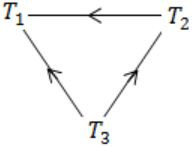Hence, S1 is conflict equivalent to S2, but not to S3.
 Question 64

A 1Mbps satellite link connects two ground stations. The altitude of the satellite is 36,504 km and speed of the signal is 3 × 108 m/s. What should be the packet size for a channel utilization of 25% for a satellite link using go-back-127 sliding window protocol? Assume that the acknowledgment packets are negligible in size and that there are no errors during communication.

 A 120 bytes B 60 bytes C 240 bytes D 90 bytes
Computer-Networks       Sliding-Window-Protocol
Question 64 Explanation:
Time to reach satellite = 36504000/3×108 = 0.121685
RTT = 4×Time to reach satellite (S1→S, S→S2, S2→S, S→S1)∴ RTT = 0.48
Efficiency = N×Tt/Tt+2Tp
= N×Tt/Tt+RTT
0.25 = 127×Tt/Tt+0.48
0.25Tt + 0.25 × 0.48 = 127Tt
0.25 × 0.48 = 126.5Tt
0.25 × 0.48 × 106/126.5 = L
L = 952 bit ≈ 120 byte
 Question 65

The minimum frame size required for a CSMA/CD based computer network running at 1 Gbps on a 200 m cable with a link speed of 2 × 10m/s is

 A 125 bytes B 250 bytes C 500 bytes D None of these
Computer-Networks       Ethernet
Question 65 Explanation:
For CSMA/CD protocol to work, the transmission time of the frame must be more than minimum value. This minimum value is the RTT.
So,
Tt ≥ 2 × Tp
L/B ≥ 2 × Tp
L ≥ 2 × Tp × B
L ≥ 2 × 200/(2×108) × 109
L ≥ 2000 bits
L ≥ 250 Bytes
 Question 66

Data transmitted on a link uses the following 2D parity scheme for error detection: Each sequence of 28 bits is arranged in a 4×7 matrix (rows r0 through r3, and columns d7 through d1) and is padded with a column d0 and row r4 of parity bits computed using the Even parity scheme. Each bit of column d0 (respectively, row r4) gives the parity of the corresponding row (respectively, column). These 40 bits are transmitted over the data link.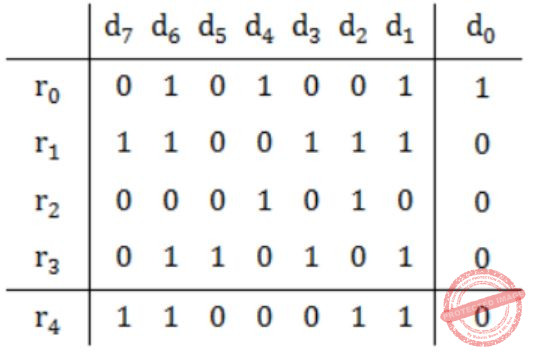The table shows data received by a receiver and has n corrupted bits. What is the minimum possible value of n?

 A 1 B 2 C 3 D 4
Computer-Networks       Error-Detection
Question 66 Explanation:Here, we need to change minimum 3-bits, and by doing it we get correct parity column wise and row wise (correction marked by boxed number).
 Question 67

Two popular routing algorithms are Distance Vector(DV) and Link State (LS) routing. Which of the following are true?

• (S1) Count to infinity is a problem only with DV and not LS routing

• (S2) In LS, the shortest path algorithm is run only at one node

• (S3) In DV, the shortest path algorithm is run only at one node

(S4) DV requires lesser number of network messages than LS
 A S1, S2 and S4 only B S1, S3 and S4 only C S2 and S3 only D S1 and S4 only
Computer-Networks       Routing
Question 67 Explanation:
→ Count to infinity problem only exist into the DVR.
→ In LSR shortest path is calculated at each every router. (B) is wrong.
→ In DVR also shortest path is calculated at each and every router. (C) is wrong.
→ Since DVR is based upon local knowledge whereas LSR is based upon global knowledge.
 Question 68

Which of the following statements are TRUE?

• (S1) TCP handles both congestion and flow control

• (S2) UDP handles congestion but not flow control

• (S3) Fast retransmit deals with congestion but not flow control

(S4) Slow start mechanism deals with both congestion and flow control
 A S1, S2 and S3 only B S1 and S3 only C S3 and S4 only D S1, S3 and S4 only
Computer-Networks       Congestion-Control
Question 68 Explanation:
S1:
TCP handles both congestion and flow control ⇒ True.
S2:
UDP handles congestion but not flow control ⇒ False, because UDP neither handles congestion control nor flow control.
S3:
Fast retransmits deals with congestion but not flow control ⇒ True.
S4:
Slow start mechanism deals with both congestion and flow control ⇒ False, because it has nothing to do with flow control. Flow control is taken care by Advertisement window.
 Question 69

The three way handshake for TCP connection establishment is shown below.Which of the following statements are TRUE? (S1) Loss of SYN + ACK from the server will not establish a connection (S2) Loss of ACK from the client cannot establish the connection (S3) The server moves LISTEN → SYN_RCVD → SYN_SENT → ESTABLISHED in the state machine on no packet loss (S4) The server moves LISTEN → SYN_RCVD → ESTABLISHED in the state machine on no packet loss.

 A S2 and S3 only B S1 and S4 C S1 and S3 D S2 and S4
Computer-Networks       TCP
Question 69 Explanation:
S1 → True.
S2 → False, because if after ACK client immediately sends data then everything goes on without worry.
S3 → False.
S4 → True.
 Question 70

The total number of keys required for a set of n individuals to be able to communicate with each other using secret key and public key crypto-systems, respectively are:

 A n(n-1) and 2n B 2n and n(n-1)/2 C n(n-1)/2 and 2n D n(n-1)/2 and n
Computer-Networks       Network-Security
Question 70 Explanation:
For private key crypto, a key used for encryption as well as decryption. So, no. of keys required for n individuals is same as no. of communication link between any two individuals which is
nC2 = n(n-1)/2
In case of public key, each sender has its own public key as well as private key. So, no. of keys are 2n.
 Question 71

A Binary Search Tree (BST) stores values in the range 37 to 573. Consider the following sequence of keys.

```I. 81, 537, 102, 439, 285, 376, 305
II. 52, 97, 121, 195, 242, 381, 472
III. 142, 248, 520, 386, 345, 270, 307
IV. 550, 149, 507, 395, 463, 402, 270```

Suppose the BST has been unsuccessfully searched for key 273. Which all of the above sequences list nodes in the order in which we could have encountered them in the search?

 A II and III only B I and III only C III and IV only D III only
Data-Structures       Binary-Trees
Question 71 Explanation:Question 72

A Binary Search Tree (BST) stores values in the range 37 to 573. Consider the following sequence of keys.

``` I. 81, 537, 102, 439, 285, 376, 305
II. 52, 97, 121, 195, 242, 381, 472
III. 142, 248, 520, 386, 345, 270, 307
IV. 550, 149, 507, 395, 463, 402, 270```

Which of the following statements is TRUE?

 A I, II and IV are inorder sequences of three different BSTs B I is a preorder sequence of some BST with 439 as the root C II is an inorder sequence of some BST where 121 is the root and 52 is a leaf D IV is a postorder sequence of some BST with 149 as the root
Data-Structures       Binary-Trees
Question 72 Explanation:
A) Incorrect because I & IV are not in ascending order. (Inorder sequence of BST is in increasing order).
B) False because if 439 is root then it should be first element in preorder.
C) This is correct.
D) False because if 149 is root, then it should be last element in postorder.
 Question 73

A Binary Search Tree (BST) stores values in the range 37 to 573. Consider the following sequence of keys.

``` I. 81, 537, 102, 439, 285, 376, 305
II. 52, 97, 121, 195, 242, 381, 472
III. 142, 248, 520, 386, 345, 270, 307
IV. 550, 149, 507, 395, 463, 402, 270```

How many distinct BSTs can be constructed with 3 distinct keys?

 A 4 B 5 C 6 D 9
Data-Structures       Binary-Trees
Question 73 Explanation:
Formula:
2nCn/n+1 = 6C3/7 = 5
 Question 74

Consider the following relational schema:

```Student (school-id, sch-roll-no, sname, saddress)
School (school-id, sch-name, sch-address, sch-phone)
Enrolment(school-id, sch-roll-no, erollno, examname)
ExamResult(erollno, examname, marks)```

What does the following SQL query output?

```SELECT  sch-name, COUNT (*)
FROM    School C, Enrolment E, ExamResult R
WHERE   E.school-id = C.school-id
AND
E.examname = R.examname AND E.erollno = R.erollno
AND
R.marks = 100 AND S.school-id IN (SELECT school-id
FROM student
GROUP BY school-id
HAVING COUNT (*) > 200)
GROUP By school-id```
 A for each school with more than 200 students appearing in exams, the name of the school and the number of 100s scored by its students B for each school with more than 200 students in it, the name of the school and the number of 100s scored by its students C for each school with more than 200 students in it, the name of the school and the number of its students scoring 100 in at least one exam D nothing; the query has a syntax error
Database-Management-System       SQL
Question 74 Explanation:
If select clause consist of aggregate and non-aggregate columns, all non-aggregate columns in the select clause must appear in Group By clause. But in this Group By clause consists school-id instead of school-name.
 Question 75

Consider the following relational schema:

```Student (school-id, sch-roll-no, sname, saddress)
School (school-id, sch-name, sch-address, sch-phone)
Enrolment(school-id, sch-roll-no, erollno, examname)
ExamResult(erollno, examname, marks)```

Consider the following tuple relational calculus query:

If a student needs to score more than 35 marks to pass an exam, what does the query return?
 A The empty set B schools with more than 35% of its students enrolled in some exam or the other C schools with a pass percentage above 35% over all exams taken together D schools with a pass percentage above 35% over each exam
Database-Management-System       Relational-Calculus
Question 75 Explanation:
Query having the division with
{ x | x ∈ Enrollment ∧ x . school-id = t } | * 100 > 35 }
This is school with enrollment % is 35 or above.
As we are actually taking percentage of
(Total count in which a student has passed from a particular school)/(Total exams taken by all student combined)
Eg: if A passed in 3 and B passed in 4 and they both took 5-5 exam each. Then it is (7/10)
 Question 76

A binary tree with n > 1 nodes has n1, n2 and n3 nodes of degree one, two and three respectively. The degree of a node is defined as the number of its neighbors. n3 can be expressed as

 A n1 + n2 - 1 B n1 - 2 C [((n1 + n2)/2)] D n2 - 1
Data-Structures       Binary-Trees
Question 76 Explanation: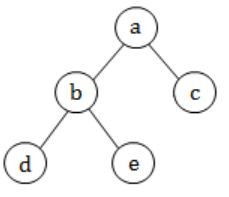n1 = 3
n2 = 1
n3 = 1
So, option (B) satisfies.
 Question 77

A binary tree with n > 1 nodes has n1, n2 and n3 nodes of degree one, two and three respectively. The degree of a node is defined as the number of its neighbors. Starting with the above tree, while there remains a node v of degree two in the tree, add an edge between the two neighbors of v and then remove v from the tree. How many edges will remain at the end of the process?

 A 2 * n1 – 3 B n2 + 2 * n1 – 2 C n3 – n2 D n2 + n1 – 2
Data-Structures       Binary-Trees
Question 77 Explanation:n1 = 3
n3 = 1
So, option (A) satisfies.
 Question 78

A CFG G is given with the following productions where S is the start symbol, A is a non-terminal and a and b are terminals.

```S → aS∣A
A → aAb∣bAa∣ϵ```
Which of the following strings is generated by the grammar above?
 A aabbaba B aabaaba C abababb D aabbaab
Theory-of-Computation       Contest-Free-Grammar
Question 78 Explanation:
S→aS
S→aA
S→aaAb
S→aabAab
S→aabbAaab
S→aabbaab
 Question 79

A CFG G is given with the following productions where S is the start symbol, A is a non-terminal and a and b are terminals.

```S → aS∣A
A → aAb∣bAa∣ϵ```
For the correct answer in Q75, how many steps are required to derive the string and how many parse trees are there?
 A 6 and 1 B 6 and 2 C 7 and 2 D 4 and 2
Theory-of-Computation       Contest-Free-Grammar
Question 79 Explanation:
S→aS
S→aA
S→aaAb
S→aabAab
S→aabbAaab
S→aabbaab
⇒ 6 steps are requiredOnly 1 parse tree is there.
 Question 80

Consider a computer with a 4-ways set-associative mapped cache of the following characteristics: a total of 1 MB of main memory, a word size of 1 byte, a block size of 128 words and a cache size of 8 KB. The number of bits in the TAG, SET and WORD fields, respectively are:

 A 7, 6, 7 B 8, 5, 7 C 8, 6, 6 D 9, 4, 7
Computer-Organization       Cache
Question 80 Explanation:
No. of cache blocks = 8KB/128 = 213/27 = 26
No. of sets in cache = 26/22 = 24
So no. of set bits = 4
Size of block = 128 words = 128 B = 27
So no. of word bit = 7
So tag bits = 20 - 4 - 7 = 9
 Question 81

Consider a computer with a 4-ways set-associative mapped cache of the following characteristics: a total of 1 MB of main memory, a word size of 1 byte, a block size of 128 words and a cache size of 8 KB. While accessing the memory location 0C795H by the CPU, the contents of the TAG field of the corresponding cache line is

 A 000011000 B 110001111 C 00011000 D 110010101
Computer-Organization       Cache
Question 81 Explanation:
As we have found in earlier question that first 9 bits is tag bits.
So lets first convert given Hexadecimal no. into binary number,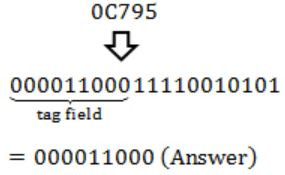Question 82

Consider the code fragment written in C below :

```void f (int n)
{
if (n <=1)  {
printf ("%d", n);
}
else {
f (n/2);
printf ("%d", n%2);
}
}```

What does f(173) print?

 A 010110101 B 010101101 C 10110101 D 10101101
Programming       C-Programming
Question 82 Explanation:So, after traversing the tree we get:
10101101
 Question 83

Consider the code fragment written in C below :

```void f (int n)
{
if (n <= 1)  {
printf ("%d", n);
}
else {
f (n/2);
printf ("%d", n%2);
}
}```

Which of the following implementations will produce the same output for f(173) as the above code?

```P1
void f (int n)
{
if (n/2)  {
f(n/2);
}
printf ("%d", n%2);
}

P2
void f (int n)
{
if (n <=1)  {
printf ("%d", n);
}
else {
printf ("%d", n%2);
f (n/2);
}
}```
 A Both P1 and P2 B P2 only C P1 only D Neither P1 nor P2
Programming       C-Programming
Question 83 Explanation:
P1 prints same as the original program.
But P2 prints the reverse of original sequence printed by original program.
 Question 84

Host X has IP address 192.168.1.97 and is connected through two routers R1 and R2 to an­other host Y with IP address 192.168.1.80. Router R1 has IP addresses 192.168.1.135 and 192.168.1.110. R2 has IP addresses 192.168.1.67 and 192.168.1.155. The netmask used in the network is 255.255.255.224. Given the information above, how many distinct subnets are guaranteed to already exist in the network?

 A 1 B 2 C 3 D 6
Computer-Networks       IP-Address
Question 84 Explanation:
Simply, Bitwise AND the bits of fourth octet for each of the following IP address with fourth octet of subnet mask. You will get only
XX.XX.XX.96, XX.XX.XX.64 and XX.XX.XX.128.
 Question 85

Host X has IP address 192.168.1.97 and is connected through two routers R1 and R2 to an­other host Y with IP address 192.168.1.80. Router R1 has IP addresses 192.168.1.135 and 192.168.1.110. R2 has IP addresses 192.168.1.67 and 192.168.1.155. The netmask used in the network is 255.255.255.224. Which IP address should X configure its gateway as?

 A 192.168.1.67 B 192.168.1.110 C 192.168.1.135 D 192.168.1.155
Computer-Networks       IP-Address
Question 85 Explanation:
X must be able to reach the gateway using the net mask.
Subnet no. of host X is,Now, the gateway must also have the same subnet number.
Let's take IP 192.168.1.110 of R1,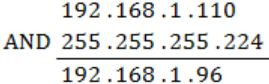and hence this can be used by X.
There are 85 questions to complete.
PHP Code Snippets Powered By : XYZScripts.com
error: Content is protected !!